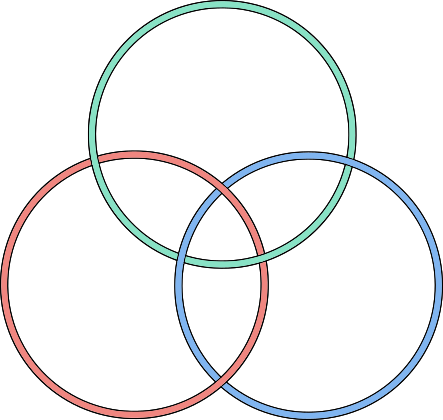Celtic knots, or interlace, are a beautiful, ancient art form with interesting symmetries and topological properties:

It turns out that you can follow a recipe to turn any planar graph into its corresponding knotwork, or vice-versa. (You can follow this recipe, the Mercat procedure, by hand; alternatively, it's precise enough that you can implement it as a computer program.)

With this recipe in hand, I began seriously wondering what some of my favorite and familiar graphs would look like as knotwork. What about the triforce symbol? Or a square? What about a cube? Oh! And what about the rest of the platonic solids as well?

Yes, that was a good challenge. The platonic solids are fun to try to visualize (I hadn't learned how to effectively visualize yarn wrapping around 3D shapes, so I had to try several ways of drawing them in two-dimensional flatland; see below.). After much trial and error, I got the following glorious series of pictures:From left to right, knotwork corresponding to the tetrahedron, cube, octahedron, dodecahedron, and icosahedron.

As you can see here,

1. Each knotwork consists of interlocked circles (3 for the tetrahedron; 4 for the cube and octahedron; 6 for the dodecahedron and icosahedron).
2. Corresponding pairs of dual shapes have the same number of circles (cube-octahedron, dodecahedron-icosahedron). They vary based on how the circles are arranged; e.g. whether there's a central circle.
3. Each knotwork has various symmetries including rotational and reflective, as well as the over-under pattern of string crossings.
4. The overlapping circles create regions with recognizable shapes. For example, the icosahedron's knotwork is made up of five-sided and three-sided regions (with a pentagon in the middle). Its dual, the dodecahedron, is made of the same shapes, but with a triangle in the middle. You can see these shapes more clearly when the regions are colored in a checkerboard pattern (see below). The cube and octahedron include four-sided regions.
5. There are other patterns if you look—for example, how many stars can you spot in the icosahedron and dodecahedron knotwork below?

Mathematical remark: To count the number of threads, you can use the formula: $$|T(−1,−1)|=2^c−1$$ where T is the Tutte polynomial of the planar graph, and c is the number of threads. Incidentally, because the dual of a graph has the same Tutte polynomial with x and y exchanged, this implies a full generalization of the result we discovered here: every graph has the same number of knotwork strands as its dual.

Mathematical remark: As shown in the checkerboard-shaded pictures, the knotwork for each polyhedron divides space into two kinds of shape, e.g. triangles and pentagons. The two shapes are apparently directly related to the Schlaffi symbol for the polyhedron—that is, the shape of each face, and the number of faces meeting at each vertex.

## 1 How I generated these

To generate this knotwork, you start with one of the figures such as the icosahedron:

You want to stretch it so that you can draw it on a flat piece of paper without any of the lines crossing. In this particular case, you can take the back-face triangle and pull all of its vertices out from behind the icosahedron. The result is a planar graph:

Now we can apply the Mercat procedure to draw threads that cross in pairs at the midpoint of every edge in the graph. The result is a bit messy, but if you color in each of the threads in a different color, you see that the knotwork is made up of simple (non-self-intersecting) closed loops.

To straighten out the wobby loops into perfect circles, all I did was arrange some colored circles by trial and error into positions that had the same symmetry as the knotwork. I then checked whether my circles had the same crossings as the knotwork, and made the same shaped regions (triangles, pentagons). The result is as you've seen, and you can repeat this process for any of the other polyhedra: